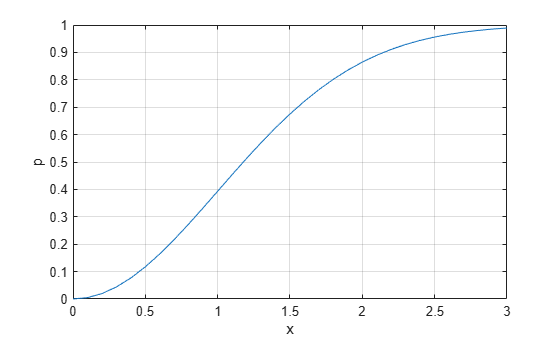raylcdf

Rayleigh cumulative distribution function

Syntax

p = raylcdf(x,b)
p = raylcdf(x,b,'upper')

Description

p = raylcdf(x,b) returns the Rayleigh cdf at each value in x using the corresponding scale parameter, b. x and b can be vectors, matrices, or multidimensional arrays that all have the same size. A scalar input for x or b is expanded to a constant array with the same dimensions as the other input.

p = raylcdf(x,b,'upper') returns the complement of the Rayleigh cdf at each value in x, using an algorithm that more accurately computes the extreme upper tail probabilities.

The Rayleigh cdf is

$y=F\left(x|b\right)={\int }_{0}^{x}\frac{t}{{b}^{2}}{e}^{\left(\frac{-{t}^{2}}{2{b}^{2}}\right)}dt$

Examples

collapse all

Compute the cdf of a Rayleigh distribution with parameter B = 1.

x = 0:0.1:3;
p = raylcdf(x,1);

Plot the cdf.

figure;
plot(x,p)References

 Evans, M., N. Hastings, and B. Peacock. Statistical Distributions. Hoboken, NJ: Wiley-Interscience, 2000. pp. 134–136.